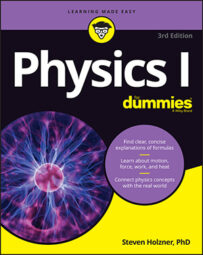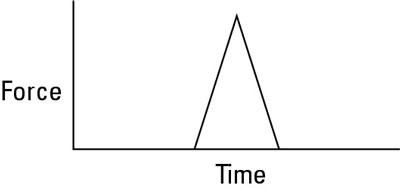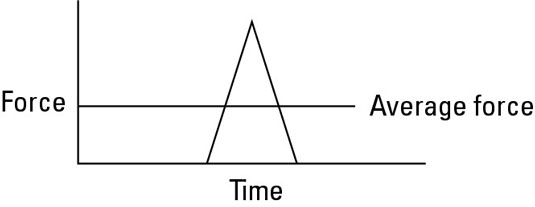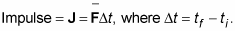##### Physics I For DummiesIn physics terms, impulse tells you how much the momentum of an object will change when a force is applied for a certain amount of time. Say, for example, that you’re shooting pool. Instinctively, you know how hard to tap each ball to get the results you want. The nine ball in the corner pocket? No problem — tap it and there it goes. The three ball bouncing off the side cushion into the other corner pocket? Another tap, this time a little stronger.Examining force versus time gives you the impulse you apply on objects.

The taps you apply are called impulses. Take a look at what happens on a microscopic scale, millisecond by millisecond, as you tap a pool ball. The force you apply with your cue appears in the figure. The tip of each cue has a cushion, so the impact of the cue is spread out over a few milliseconds as the cushion squashes slightly. The impact lasts from the time when the cue touches the ball, ti, to the time when the ball loses contact with the cue, tf. As you can see from the figure, the force exerted on the ball changes during that time; in fact, it changes drastically, and figuring out what the force was doing at any 1 millisecond would be hard without some fancy equipment.

Because the pool ball doesn’t come with any fancy equipment, you have to do what physicists normally do, which is to talk in terms of the average force over time. You can see what that average force looks like in the following figure. Speaking as a physicist, you say that the impulse — or the tap — that the pool cue provides is the average force multiplied by the time that you apply the force.The average force over a time interval depends on the values the force has over that time.

Here’s the equation for impulse:Note that this equation is a vector equation, meaning it deals with both direction and magnitude. Impulse, J, is a vector, and it’s in the same direction as the average force (which itself may be a net vector sum of other forces).

You get impulse by multiplying a quantity with units of newtons by a quantity with units of seconds, so the units of impulse are newton-seconds in the MKS (meter-kilogram-second) system and pound-seconds in the FPS (foot-pound-second) system.